Smartick is an advanced online program that teaches kids math and coding in only 15 min. a day

Jul11

# Geometric Figures and Straight Lines

Straight lines are key to recognizing shapes that we often see in the real world: geometric figures.

In today’s post, we will classify geometric figures formed from straight lines and think about where we can find them.

### Two Straight Lines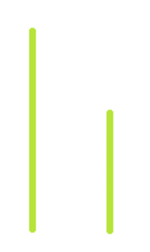Can you make a figure with two straight lines?Well, of course, you can! However, we do not call this figure a geometrical figure. In order to be a geometrical figure, it must be “closed.”  That is to say, if we put a ball inside the figure, it could not escape. In the figure we have made, the ball could escape!If you try to move the two lines, you will see that in no way can it be closed. You could take two straight objects, for example, two chopsticks, and try.

### Basic Geometric Figures

Let’s now try it with three straight lines…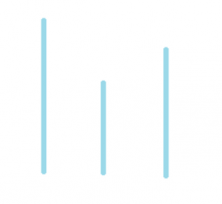That’s it! Now you can make a figure that the ball cannot escape.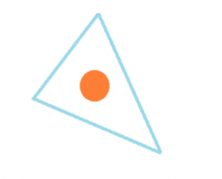Each of the straight lines we used now act as a part of the figure.

We will call each straight line a side of the figure. So, this figure has three sides and is called a triangle. When we say “triangle” we are either referring to how to arrange the three lines or the enclosing space.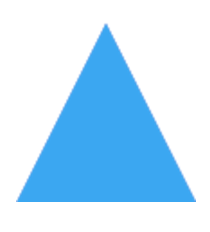Can you imagine where we can find triangles around us? I found some in Smartick, let’s see if you recognize them!

###4-Sided Geometric Figures

Let’s see what we can do with 4 straight lines…We could make, for example,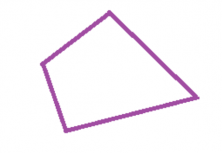We call these figures quadrilaterals. There are some very special quadrilaterals that have pairs of parallel sides. That is, there are two sides which are parallel to each other, and the other two are also. In addition, the sides that are parallel are equal. How could we make these? We get two equal sides and put them parallel to each other: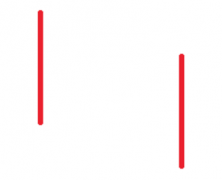How can we place the other two sides so that they are also parallel? That’s it! Simply by joining them together.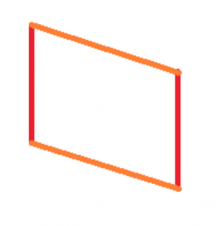At this point we have three possibilities:

• All the sides are equal, creating a diamond.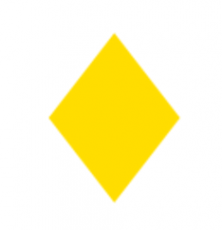Where can you find diamonds?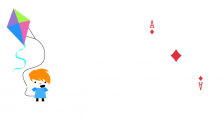I found two!

• If the four angles of the figure are right angles, then we have created a rectangle.We can find many rectangles around us … I’m sure that you can think of many!

###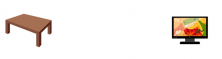• If we create a geometric shape that meets both of the above conditions and also has all equal sides and four right angles it is a square.

###Geometric Figures With More Than 4 Sides

We can keep adding all the lines we want to create different shapes with different numbers of sides. They are especially important when they have equal sides. For example, the following would be the pentagon (5-sided).We can find them, for example, in the drawing of soccer balls.And then we have the hexagon (6-sided).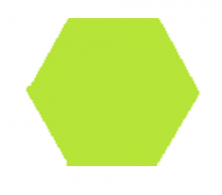We can find them … in beehives! How do bees know how to make such perfect geometric shapes?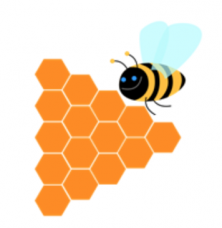And then heptagons, octagons, enneagon, nonagons … any missing? Of course! What can we do with curved lines? With curved lines, it does not make sense to classify them because they vary so much more … but I can tell you that there is a very, very important curved figure that I am sure you already know of… the circle!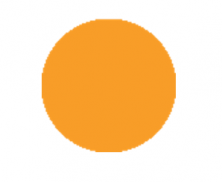We get a circle when we maintain the distance from a point. For example, with a compass, we set a point with a needle. Then, keeping the opening, we draw the circle with a pencil. You can find a lot of circles around you, on the wheels of your car or a tennis ball.If you want, you can continue learning elementary math with Smartick!